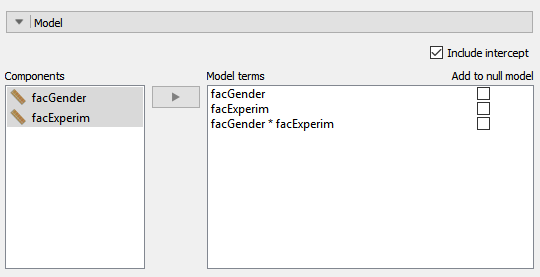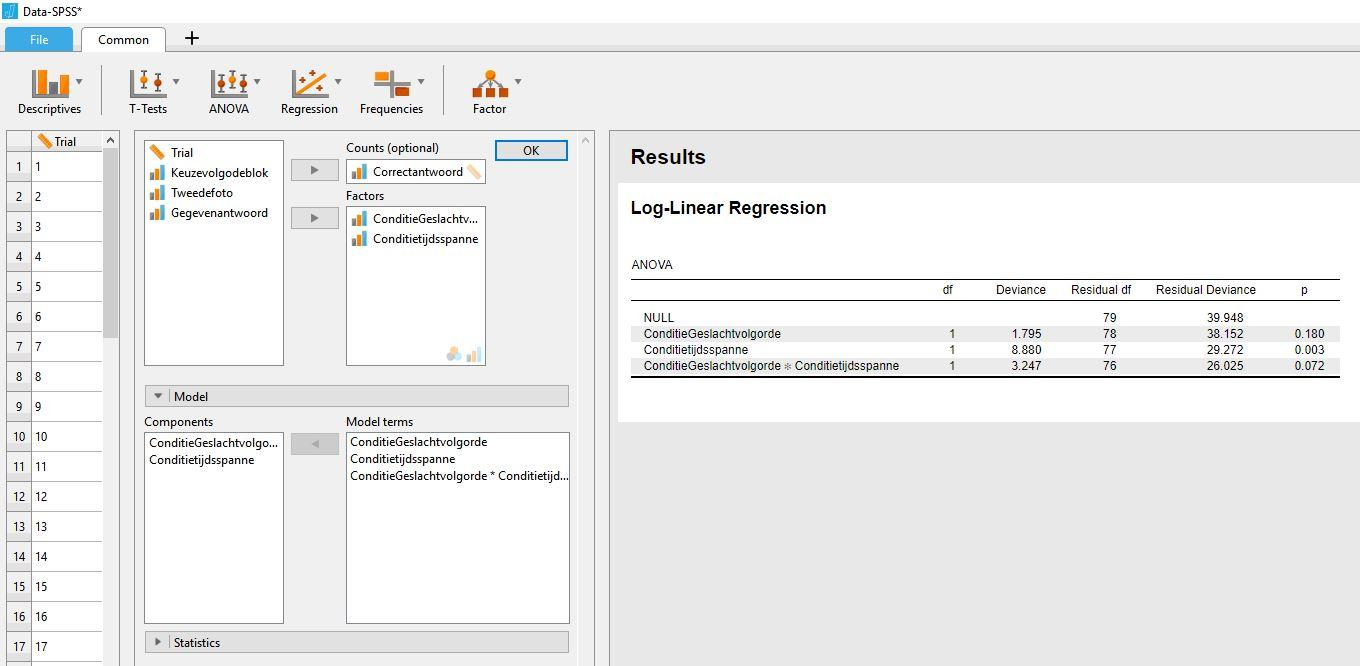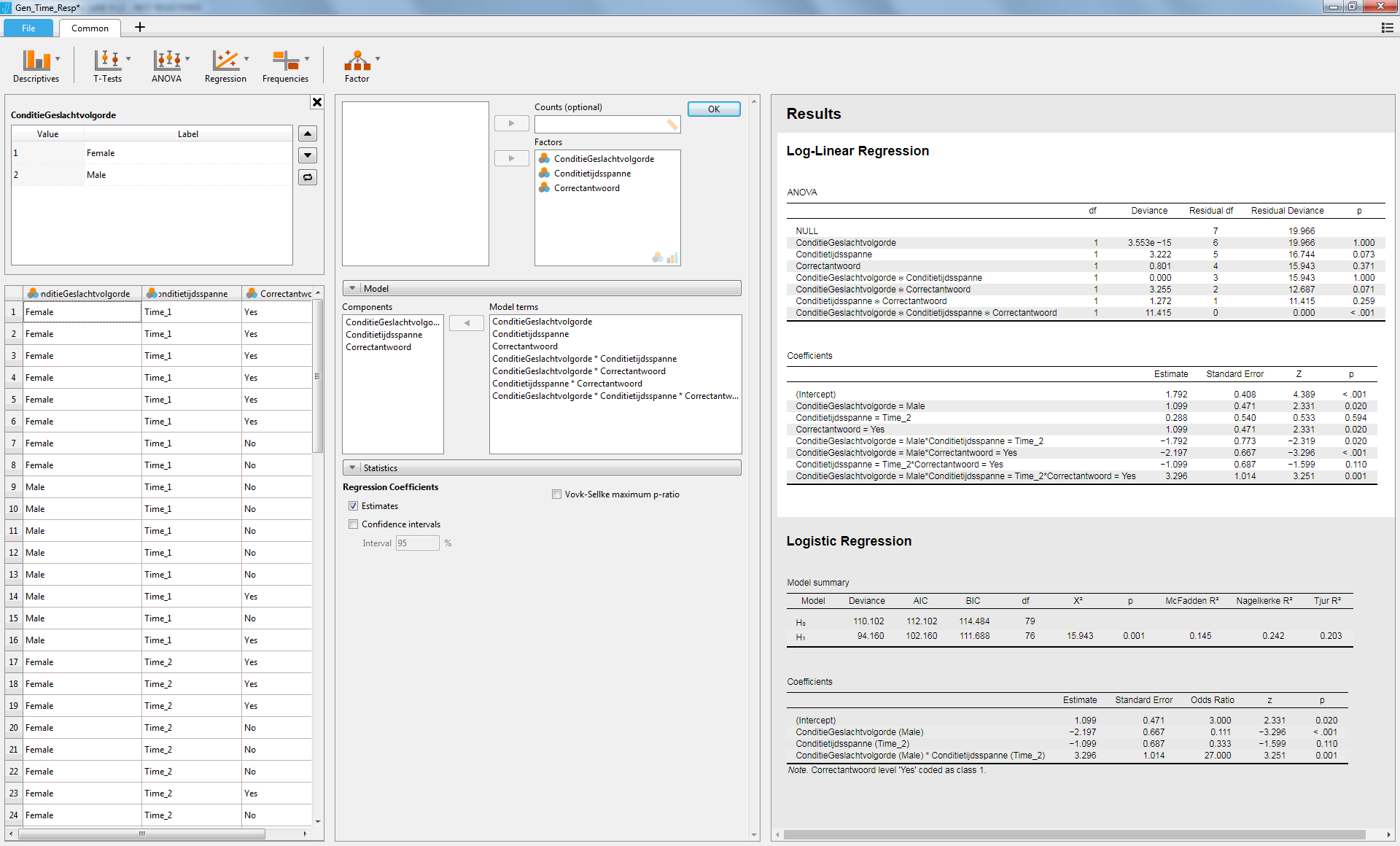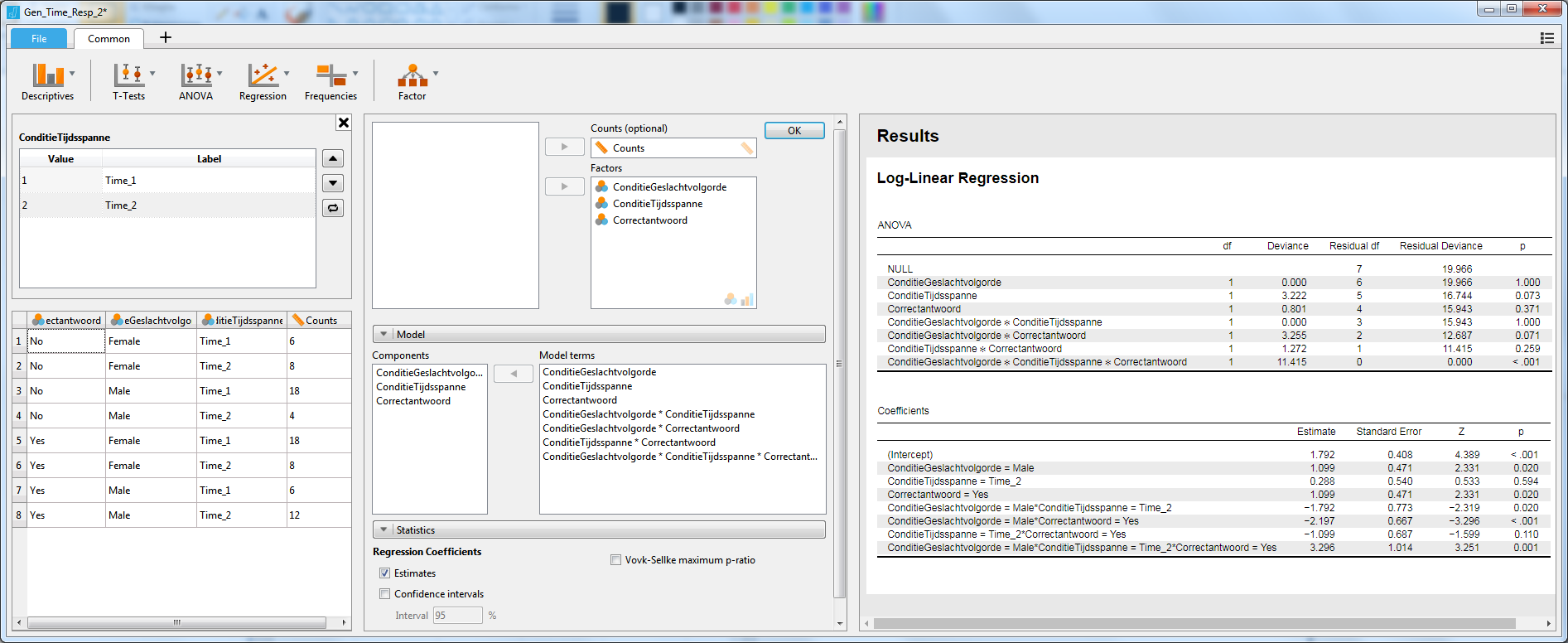#### Howdy, Stranger!

It looks like you're new here. If you want to get involved, click one of these buttons!

Supported by

# Logistic regression and interaction

edited March 2018

Hello I am testing JASP
I want to perform a logistic regression analysis on two independent variables (both have two states 0 and 1) with one dependent variable (the number of correct answers)
In SPSS I can calulate the interaction between the two independent variables
is this also possible in JASP?

• Hi Jan,

I'll forward this question to our logistic regression expert, but I think the expert will probably want to know what you mean exactly. In logistic regression, your dependent variable needs to be binary; for the predictors there is no such restriction. So it would be useful to know which y exactly you are trying to predict with which x's.

Cheers,
E.J.

• Hello E.J.
the y is correct answers with values 0 and value 1
my independent variables are also binary:
timerelation with value 1 or 2
and genderorder with values 1or 2

• Hi Jan,
since you have three dichotomous variables, you can use log-linear analysis.
In Jasp:
Frequencies -> Log-Linear Regression
and enter the three variables in the Factors box.

Cheers,
MAgoJ

• Hi Jan,

I'd like to add that you can also add interactions in a similar way to how it's done in linear regression: open the the `Model` section, select both variables together in `components` (by holding shift while selecting) and add it to `Model Terms`.

It will look somewhat like this:• Hello MAgoJ,
i am going to work that out!

• Hello evankesteren,
thank you for your suggestion!
I will work on that too

• Hello evanKesteren
something like this?• Hi Jan,
from the attached image, I understand that the variable Correctantwoord only returns 0 and 1 to indicate wrong or correct answer.
You must enter it in the Factor box, not in the Counts (optional) box.
If your data were organized as a contingency table, the counting variable to be included in the Counts box would be frequencies.
I am attaching screenshots1 for fake data, organized like yours and screenshot_2 for the same data organized as frequencies from the contingency table.

Cheers,
MAgoJ• Hello MAgoJ,
thank you very much thats clear!
i will look at my data again

• Hello MAgoJ,
works perfect thank you